# SAT II Math II : Other 3-Dimensional Geometry

## Example Questions

### Example Question #46 : 3 Dimensional Geometry

A convex polyhedron has twenty faces and thirty-six vertices. How many edges does it have?Explanation:

The number of vertices, edges, and facesof any convex polyhedron are related by By Euler's Formula: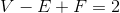Setting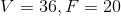and solving for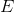: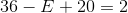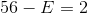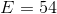The polyhedron has 54 edges.